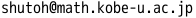Nobumichi SHUTOH Nobumichi SHUTOH Department of Mathematics, Graduate School of Science, Kobe University Division : Applied Mathematics Professor Building B, Room 214Research Field : Mathematical statistics, Missing data analysis, and High-dimensional data analysis. Research Summary : We consider the statistical hypothesis testing procedures with missing data. By obtaining Bartlett-type correction of the test statistics, we propose the testing procedures which perform better even if the sample size is small. Furthermore, we also extend the above-mentioned results to the case of elliptically contoured distributions. Primary Publications : N. Shutoh . Effect of nonnormality on tests for a mean vector with missing data under an elliptically contoured pattern-mixture model, Communications in Statistics - Theory and Methods, 50, 4448-4469. (2021) T. Kawasaki, N. Shutoh, T. Seo . On the asymptotic distribution of \$T^2\$-type statistic with two-step monotone missing data, Journal of Statistical Theory and Practice, 12, 657-668. (2018) N. Shutoh, T. Nishiyama, M. Hyodo. Bartlett correction to the likelihood ratio test for MCAR with two-step monotone sample, Statistica Neerlandica, 71, 184-199. (2017) S. Takahashi, N. Shutoh . Tests for parallelism and flatness hypotheses of two mean vectors in high-dimensional settings, Journal of Statistical Computation and Simulation, 86, 1150-1165. (2016) M. Hyodo, N. Shutoh, T. Nishiyama, T. Pavlenko (2015). Testing block-diagonal covariance structure for high-dimensional data, Statistica Neerlandica, 69, 460-482. N. Shutoh . An asymptotic approximation for EPMC in linear discriminant analysis based on monotone missing data, Journal of Statistical Planning and Inference, 142, 110-125. (2012)